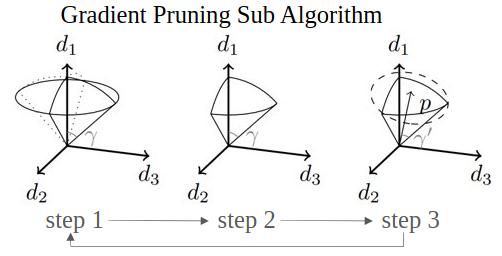# Smooth Convex Optimization Using Sub-Zeroth-Order Oracles

Mustafa O. Karabag, Cyrus Neary, and Ufuk Topcu
The AAAI Conference on Artificial Intelligence (AAAI) 2021.

PaperAbstract: We consider the problem of minimizing a smooth, Lipschitz, convex function over a compact, convex set using sub-zeroth-order oracles: an oracle that outputs the sign of the directional derivative for a given point and a given direction, an oracle that compares the function values for a given pair of points, and an oracle that outputs a noisy function value for a given point. We show that the sample complexity of optimization using these oracles is polynomial in the relevant parameters. The optimization algorithm that we provide for the comparator oracle is the first algorithm with a known rate of convergence that is polynomial in the number of dimensions. We also give an algorithm for the noisy-value oracle that incurs sublinear regret in the number of queries and polynomial regret in the number of dimensions.